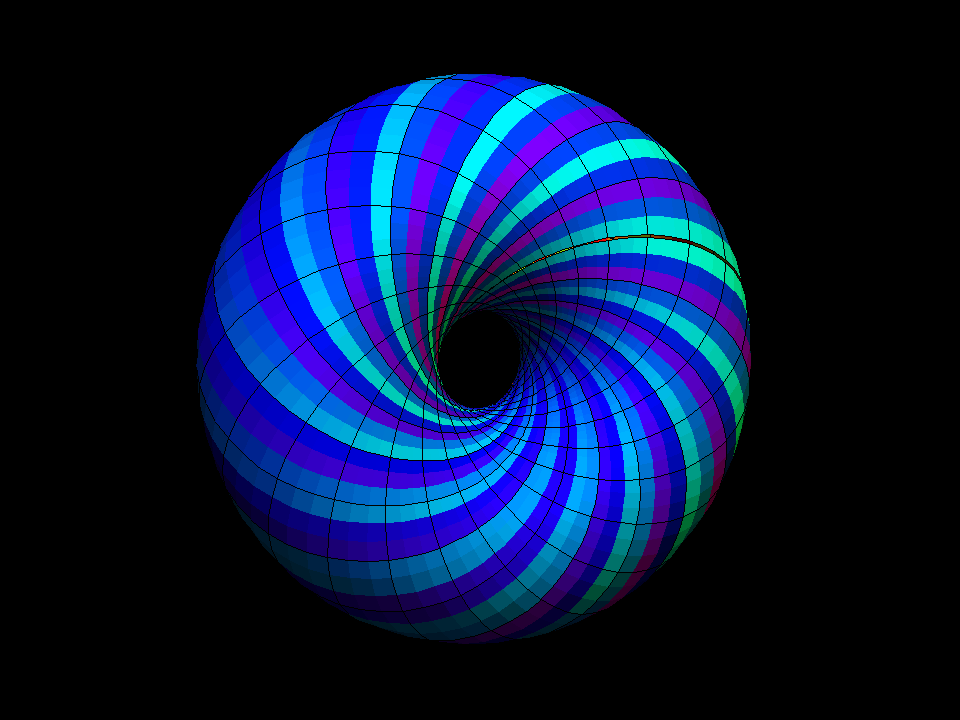# Clifford TorusThe Clifford Torus is the torus S^1/sqrt(2) x S^1/sqrt(2) in R^2 x R^2 = R^4. It actually lies in the unit sphere S^3 from where it can stereographically be projected into R^3. The animation shows how projections from different points of R^3 look. When the projection center is on the Clifford Torus then the image looks like a plane with a handle.clifford torus in out 001

Interchanging zero and infinity in R^3 by the inversion map

`(x,y,z) ⟶ (x,y,z)/(x^2+y^2+z^2)`

turns a torus which is centered at zero inside out. This can be done continuously, if we think of the torus in R^3 as the stereographic image of a torus in S^3. In R^3 we can rotate a plane around one of its lines by 180 degrees - so that we can see the other side of the plane. Similarly, in S^3 we can rotate a Clifford torus around one of the great circles on it also by 180 degrees and this exchanges the two sides of the torus. If we follow this rotation by stereographic projection into R^3, then we can actually see it. The rotating torus in S^3 has to pass through the center of the stereographic projection - at this moment the projection into R^3 looks like a plane with a handle.A torus can be parametrized in such a way that one set of parameter lines are 3-2-knots. (In the other direction we only go until we meet the knot again. In other words we rap a 3-2-band around the torus so that the ends of this band meet.) In this animation, if we look from the orange side then the color bands and the gap are 3-2-knots; from the blue side we see 2-3-knots. We use the same conformal rotation as before to turn the torus inside out and watch 3-2-knots deform into 2-3-knots.